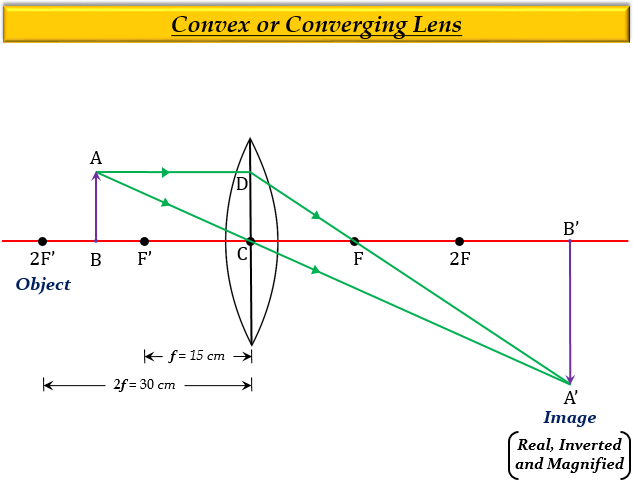# In order to obtain a real image twice the size of the object with a convex lens of focal length 15 cm, the object distance should be:(a) more than 5 cm but less than 10 cm (b) more than 10 cm but less than 15 cm(c) more than 15 cm but less than 30 cm (d) more than 30 cm but less than 60 cm

(c) More than 15 cm but less than 30 cm

Explanation

In order to obtain a real image twice the size of the object with a convex lens of focal length 15 cm, the object distance should be more than 15 cm but less than 30 cm, because, in a convex lens when an object is placed between $F$ and $2F$, the image formed is real and enlarged.

Here, $F'$ is 15cm, then $2F'$ will be 30cm, therefore the object must be placed between 15cm and 30cm.Updated on: 10-Oct-2022

419 Views